# Appearance of a Node in Blazor Diagram Component

25 Aug 202324 minutes to read

The appearance of a node can be customized by changing its Fill, StrokeDashArray, StrokeColor, StrokeWidth, and Shadow properties. The IsVisible property of the node indicates whether the node is visible or not.

The following code shows how to customize the appearance of the shape.

``````@using Syncfusion.Blazor.Diagram

<SfDiagramComponent Height="600px" Nodes="@nodes" />

@code
{
//Define diagram's nodes collection
DiagramObjectCollection<Node> nodes;

protected override void OnInitialized()
{
nodes = new DiagramObjectCollection<Node>();
// A node is created and stored in nodes array.
Node node = new Node()
{
// Position of the node
OffsetX = 250,
OffsetY = 250,
// Size of the node
Width = 100,
Height = 100,
//Sets the style for the node
Style = new ShapeStyle() { Fill = "Green", StrokeDashArray = "5,5", StrokeColor = "red", StrokeWidth = 2 },
};
}
}``````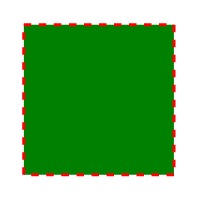NOTE

ID for each node should be unique and so it is further used to find the node at runtime and do any customization.

## How to update values in common to all nodes

Default values for all the Nodes can be set using the NodeCreating method. For example, if all nodes have the same type or property then such properties can be moved into NodeCreating method.

The following code shows how to customize the appearance of the shape.

``````@using Syncfusion.Blazor.Diagram

<SfDiagramComponent Height="600px" Nodes="@nodes" NodeCreating="@NodeCreating" />

@code
{
//Reference the diagram
SfDiagramComponent diagram;
//Define diagram's nodes collection
DiagramObjectCollection<Node> nodes;

protected override void OnInitialized()
{
nodes = new DiagramObjectCollection<Node>();
// A node is created and stored in nodes array.
Node node1 = new Node()
{
// Position of the node
OffsetX = 250,
OffsetY = 250,
// Shape of the Node
Shape = new BasicShape() { Type = NodeShapes.Basic, Shape = NodeBasicShapes.Rectangle }
};
Node node2 = new Node()
{
// Position of the node
OffsetX = 100,
OffsetY = 100,
// Shape of the Node
Shape = new BasicShape() { Type = NodeShapes.Basic, Shape = NodeBasicShapes.Ellipse }
};
}
//Method to define nodecreating event
private void NodeCreating(IDiagramObject obj)
{
Node node = obj as Node;
node.Style = new ShapeStyle() { Fill = "#6495ED" };
// Size of the node
node.Width = 100;
node.Height = 100;
}
}``````

## How to update template for the nodes

You can define node style using template in NodeTemplate at tag level. If you want to define separate template for each node, differentiate the nodes by the ID property. The following code explains how to define template for a node.

``````@using Syncfusion.Blazor.Diagram

<SfDiagramComponent @ref="diagram" Width="1200px" Height="1000px" Nodes="@nodes">
<DiagramTemplates>
<NodeTemplate>
@{
<style>
th {
}

td {
}

.c1 {
background: transparent
}

.c2 {
background: transparent
}

.c3 {
background: transparent
}

.c4 {
background: transparent
}
</style>
<div id="node0_content_html_element" class="foreign-object" style="height: 164px; width: 137px; left: 0px; top: 0px; position: absolute; transform: scale(1, 1) rotate(0deg); pointer-events: all; visibility: visible; opacity: 1;">
<div style="height: 100%; width: 100%;">
<table style="width:100%;">
<tbody>
<tr><th class="c1">ID</th><th class="c2">Offset</th></tr>
<tr><td class="c1">node0</td><td class="c2">100</td></tr>
</tbody>
</table>
<input type="button" value="Button2" @onclick="@OnClick" />
</div>
</div>
}
</NodeTemplate>
</DiagramTemplates>
</SfDiagramComponent>

@code
{
SfDiagramComponent diagram;
DiagramObjectCollection<Node> nodes;

protected override void OnInitialized()
{
nodes = new DiagramObjectCollection<Node>();
Node node = new Node()
{
Width = 137,
Height = 64,
OffsetX = 300,
OffsetY = 100,
ID = "html1",
Shape = new Shape() { Type = NodeShapes.HTML },
};
}

public void OnClick()
{
nodes.BackgroundColor = "cornflowerblue";
StateHasChanged();
}
}``````

NOTE

In the above example, node’s background color is updated using the click event of the button defined in the template.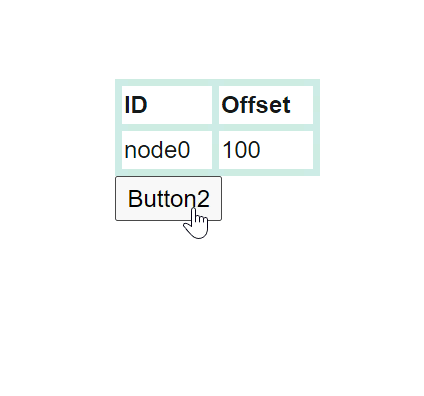## How to update style for nodes in common

The SetNodeTemplate method of diagram allows you to define the style for the Node. The following code demonstrates how to set different styles for different nodes through the SetNodeTemplate method.

``````@using Syncfusion.Blazor.Diagram
@using System.Collections.ObjectModel

@* Initialize Diagram *@
<SfDiagramComponent Height="600px" Nodes="@nodes" SetNodeTemplate="@SetNodeTemplate" />

@code
{
// Initialize node collection with node.
DiagramObjectCollection<Node> nodes;

protected override void OnInitialized()
{
nodes = new DiagramObjectCollection<Node>();
Node node1 = new Node()
{
ID = "node1",
// Size of the node.
Height = 100,
Width = 100,
// Position of the node.
OffsetX = 100,
OffsetY = 100,
};
Node node2 = new Node()
{
ID = "node6",
// Size of the node.
Height = 510,
Width = 202,
// Position of the node.
OffsetX = 300,
OffsetY = 100,
};
}

private CommonElement SetNodeTemplate(IDiagramObject node)
{
if ((node as Node).ID == "node6")
{
StackPanel table = new StackPanel();
table.Style = new ShapeStyle() { Fill = "#e6e0eb", StrokeColor = "#e6e0eb" };
table.Orientation = Orientation.Horizontal;
StackPanel column1 = new StackPanel();
column1.Style = new ShapeStyle() { Fill = "#6F409F", StrokeColor = "#6F409F" };
column1.Margin = new DiagramThickness() { Bottom = 10, Left = 10, Right = 10, Top = 10 };
column1.Padding = new DiagramThickness() { Bottom = 10, Left = 10, Right = 10, Top = 10 };
column1.Children = new ObservableCollection<CommonElement>();

StackPanel column2 = new StackPanel();
column2.Margin = new DiagramThickness() { Bottom = 10, Left = 10, Right = 10, Top = 10 };
column2.Padding = new DiagramThickness() { Bottom = 10, Left = 10, Right = 10, Top = 10 };
column2.Children = new ObservableCollection<CommonElement>();
column2.Style = new ShapeStyle() { Fill = "#6F409F", StrokeColor = "#6F409F" };

StackPanel column3 = new StackPanel();
column3.Margin = new DiagramThickness() { Bottom = 10, Left = 10, Right = 10, Top = 10 };
column3.Padding = new DiagramThickness() { Bottom = 10, Left = 10, Right = 10, Top = 10 };
column3.Children = new ObservableCollection<CommonElement>();
column3.Style = new ShapeStyle() { Fill = "#6F409F", StrokeColor = "#6F409F" };

StackPanel column4 = new StackPanel();
column4.Margin = new DiagramThickness() { Bottom = 10, Left = 10, Right = 10, Top = 10 };
column4.Padding = new DiagramThickness() { Bottom = 10, Left = 10, Right = 10, Top = 10 };
column4.Children = new ObservableCollection<CommonElement>();
column4.Style = new ShapeStyle() { Fill = "#6F409F", StrokeColor = "#6F409F" };

table.Orientation = Orientation.Vertical;
table.Children = new ObservableCollection<CommonElement>() { column1, column2, column3, column4 };
return table;
}
else
{
(node as Node).Style = new ShapeStyle()
{
Fill = "#6F409F",
StrokeColor = "#6F409F",
StrokeWidth = 2
};
(node as Node).Shape = new BasicShape()
{
Type = NodeShapes.Basic,
Shape = NodeBasicShapes.Rectangle,
};
}
return null;
}

private TextElement getTextElement(string text)
{
TextElement textElement = new TextElement();
textElement.Width = 60;
textElement.Height = 20;
textElement.Content = text;
textElement.Style = new TextStyle() { Color = "white" };
return textElement;
}
}``````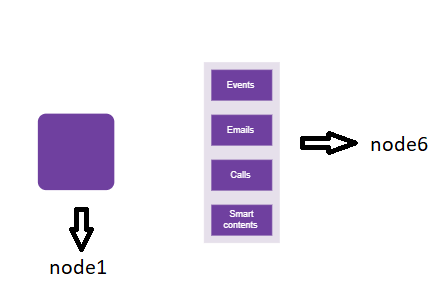## How to update shadow to a node

Diagram provides support to add Shadow effect to a node that is disabled, by default. It can be enabled with the constraints property of the node. The following code shows how to draw shadow.

``````@using Syncfusion.Blazor.Diagram

<SfDiagramComponent Height="600px" Nodes="@nodes" />

@code
{
//Define diagram's nodes collection
DiagramObjectCollection<Node> nodes;

protected override void OnInitialized()
{
nodes = new DiagramObjectCollection<Node>();
// A node is created and stored in nodes array.
Node node = new Node()
{
// Position of the node
OffsetX = 250,
OffsetY = 250,
// Size of the node
Width = 100,
Height = 100,
Style = new ShapeStyle() { Fill = "#6495ED", StrokeColor = "white" },
{
Angle = 50,
Color = "gray",
Opacity = 0.8,
Distance = 10
}
};
}
}``````### How to customize shadow to a node

The Angle, Distance, and Opacity of the shadow can be customized with the shadow property of the node. The following code example illustrates how to customize shadow.

``````@using Syncfusion.Blazor.Diagram

<SfDiagramComponent Height="600px" Nodes="@nodes" />

@code
{
DiagramObjectCollection<Node> nodes;

protected override void OnInitialized()
{
nodes = new DiagramObjectCollection<Node>();
// A node is created and stored in nodes array.
Node node = new Node()
{
// Position of the node.
OffsetX = 250,
OffsetY = 250,
// Size of the node.
Width = 100,
Height = 100,
Style = new ShapeStyle()
{
Fill = "#6495ED",
StrokeColor = "white"
},
// Custom Shadow of the node.
{
Angle = 50,
Color = "Blue",
Opacity = 0.8,
Distance = 20
}
};
}
}``````## How to apply gradient style

The Gradient property of the node allows you to define and apply the gradient effect to the node. The gradient stops property defines the color and a position, where the previous color transition ends and a new color transition starts. The gradient stop’s opacity property defines the transparency level of the region.

There are two types of gradients as follows:

### How to apply linear gradient brush

LinearGradientBrush defines a smooth transition between a set of colors (so-called stops) in a line. A linear gradient’s X1, Y1, X2, Y2 properties are used to define the position (relative to the node) of the rectangular region that needs to be painted.

``````@using Syncfusion.Blazor.Diagram

<SfDiagramComponent Height="600px" Nodes="@nodes" />

@code
{
DiagramObjectCollection<Node> nodes;

protected override void OnInitialized()
{
nodes = new DiagramObjectCollection<Node>();
// A node is created and stored in nodes array.
Node node = new Node()
{
// Position of the node.
OffsetX = 250,
OffsetY = 250,
// Size of the node.
Width = 100,
Height = 100,
Style = new ShapeStyle()
{
{
// Start point of linear gradient.
X1 = 0,
Y1 = 0,
// End point of linear gradient.
X2 = 50,
Y2 = 50,
//Sets an array of stop objects
{
new GradientStop(){ Color = "white", Offset = 0},
new GradientStop(){ Color = "#6495ED", Offset = 100}
},
}
},
};
}
}``````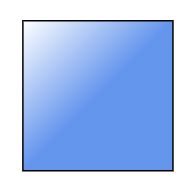RadialGradientBrush defines a smooth transition between stops on a circle. The radial gradient brush properties are used to define the position (relative to the node) of the outermost or the innermost circle of the radial gradient.

``````@using Syncfusion.Blazor.Diagram

<SfDiagramComponent Height="600px" Nodes="@nodes" />

@code
{
DiagramObjectCollection<Node> nodes;

protected override void OnInitialized()
{
nodes = new DiagramObjectCollection<Node>();
Node node = new Node()
{
// Position of the node.
OffsetX = 250,
OffsetY = 250,
// Size of the node.
Width = 100,
Height = 100,
Shape = new BasicShape()
{
Type = NodeShapes.Basic,
Shape = NodeBasicShapes.Ellipse
},
Style = new ShapeStyle()
{
Fill = "37909A#",
StrokeColor = "#024249",
{
// Center point of outer circle.
CX = 50,
CY = 50,
// Center point of inner circle.
FX = 50,
FY = 50,
{
new GradientStop() { Color = "#00555b", Offset = 45 },
new GradientStop() { Color= "#37909A", Offset= 90 }
},
}
},
};
}
}``````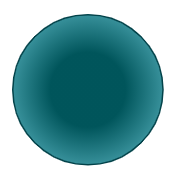The AdditionalInfo property of the node allows you to maintain additional information to the node.

The following code shows how to set the AdditionalInfo value.

``````@using Syncfusion.Blazor.Diagram

<SfDiagramComponent Height="600px" Nodes="@nodes" />

@code
{
DiagramObjectCollection<Node> nodes;

protected override void OnInitialized()
{
nodes = new DiagramObjectCollection<Node>();
Dictionary<string, object> NodeInfo = new Dictionary<string, object>();
// A node is created and stored in nodes collection.
Node node = new Node()
{
// Position of the node.
OffsetX = 250,
OffsetY = 250,
// Size of the node.
Width = 100,
Height = 100,
Style = new ShapeStyle()
{
Fill = "#6BA5D7",
StrokeColor = "white"
},
};
}
}``````

Note: You can set any type of value for the AdditionalInfo property.

## How to set ZIndex property for node

• The node’s ZIndex property specifies the stack order of the node. A node with a greater stack order is always in front of a node with a lower stack order. By default, the value is -1.

The following code illustrates how to render nodes based on the stack order.

``````@using Syncfusion.Blazor.Diagram
@using System.Collections.ObjectModel

<SfDiagramComponent Height="600px" @ref="@diagram" Nodes="@nodes" />

@code
{
SfDiagramComponent diagram;
DiagramObjectCollection<Node> nodes;

protected override void OnInitialized()
{
nodes = new DiagramObjectCollection<Node>();
// nodes are created and stored in nodes array.
Node node1 = new Node()
{
ID = "node1",
// Position of the node.
OffsetX = 250,
OffsetY = 250,
// Size of the node.
Width = 100,
Height = 100,
ZIndex = 2,
Style = new ShapeStyle()
{
Fill = "#6495ED",
StrokeColor = "white"
},
};

Node node2 = new Node()
{
ID = "node2",
// Position of the node.
OffsetX = 300,
OffsetY = 300,
// Size of the node.
Width = 100,
Height = 100,
ZIndex = 1,
Style = new ShapeStyle()
{
Fill = "#6495ED",
StrokeColor = "white"
},
};
}
}``````

## How to set pivot property for node

• Node rotation angle will be based on Pivot values which range from 0 to 1 like offset values. By default, the Pivot values are set to X= 0.5 and Y=0.5.

The following code illustrates how to set the Pivot value.

``````@using Syncfusion.Blazor.Diagram
@using System.Collections.ObjectModel

<SfDiagramComponent Height="600px" @ref="@diagram" Nodes="@nodes" />

@code
{
SfDiagramComponent diagram;
DiagramObjectCollection<Node> nodes;

protected override void OnInitialized()
{
nodes = new DiagramObjectCollection<Node>();
// A node is created and stored in nodes array.
Node node = new Node()
{
ID = "node",
// Position of the node.
OffsetX = 250,
OffsetY = 250,
// Size of the node.
Width = 100,
Height = 100,
ZIndex = 2,
Style = new ShapeStyle()
{
Fill = "#6495ED",
StrokeColor = "white"
},
// Pivot of the node.
Pivot = new DiagramPoint() { X = 0, Y = 0 }
};
}
}``````

## How to set background color for node

• The node’s BackgroundColor property is used to set the background color for node. By default, it is transparent.

The following code illustrates how to set the background color for the node.

``````@using Syncfusion.Blazor.Diagram
@using System.Collections.ObjectModel

<SfDiagramComponent Height="600px" @ref="@diagram" Nodes="@nodes" />

@code
{
SfDiagramComponent diagram;
DiagramObjectCollection<Node> nodes;

protected override void OnInitialized()
{
nodes = new DiagramObjectCollection<Node>();
// A node is created and stored in nodes array.
Node node = new Node()
{
ID = "node",
// Position of the node.
OffsetX = 250,
OffsetY = 250,
// Size of the node.
Width = 100,
Height = 100,
BackgroundColor = "red",
Shape = new BasicShape(){Shape = NodeBasicShapes.Star}
};
}
}``````

## How to check node is automatically positioned or not

• The node’s CanAutoLayout value indicates whether the node should be automatically positioned or not. Applicable if the layout option is enabled.

The following code illustrates how to set the can auto layout property for the node.

``````@using Syncfusion.Blazor.Diagram

<SfDiagramComponent Height="600px"  Nodes="@nodes" Connectors="@connectors" NodeCreating="@OnNodeCreating" ConnectorCreating="@OnConnectorCreating">
<Layout Type="LayoutType.HierarchicalTree" @bind-HorizontalSpacing="@HorizontalSpacing" @bind-VerticalSpacing="@VerticalSpacing">
</Layout>
<SnapSettings>
<HorizontalGridLines LineColor="white" LineDashArray="2,2">
</HorizontalGridLines>
<VerticalGridLines LineColor="white" LineDashArray="2,2">
</VerticalGridLines>
</SnapSettings>
</SfDiagramComponent>

@code
{
int left = 40;
int top = 50;
DiagramObjectCollection<Node> nodes = new DiagramObjectCollection<Node>();
DiagramObjectCollection<Connector> connectors = new DiagramObjectCollection<Connector>();
int HorizontalSpacing = 40;
int VerticalSpacing = 40;

private void OnNodeCreating(IDiagramObject obj)
{
Node node = obj as Node;
node.Height = 40;
node.Width = 100;
//Initialize the default node's shape style.
node.Style = new ShapeStyle() { Fill = "darkcyan", StrokeWidth = 3, StrokeColor = "Black" };
node.Annotations = new DiagramObjectCollection<ShapeAnnotation>()
{
new ShapeAnnotation
{
Content = node.Annotations.Content,
Style = new TextStyle() { Color = "white", Bold = true },
}
};
}
private void OnConnectorCreating(IDiagramObject connector)
{
(connector as Connector).Type = ConnectorSegmentType.Orthogonal;
}

protected override void OnInitialized()
{
//Initialize the node and connectors.
nodes = new DiagramObjectCollection<Node>()
{
new Node() { ID="node1", CanAutoLayout = true, Annotations = new DiagramObjectCollection<ShapeAnnotation>() { new ShapeAnnotation{Content="Steve-Ceo"} } },
new Node() { ID="node2", CanAutoLayout = true, Annotations = new DiagramObjectCollection<ShapeAnnotation>() { new ShapeAnnotation{Content="Kevin-Manager"} } },
new Node() { ID="node3", CanAutoLayout = true, Annotations = new DiagramObjectCollection<ShapeAnnotation>() { new ShapeAnnotation{Content="Peter-Manager"} } },
new Node() { ID="node4", CanAutoLayout = true, Annotations = new DiagramObjectCollection<ShapeAnnotation>() { new ShapeAnnotation{Content="Jim-CSE"} } },
new Node() { ID="node5", CanAutoLayout = true, Annotations = new DiagramObjectCollection<ShapeAnnotation>() { new ShapeAnnotation{Content="Martin-CSE"} } },
new Node() { ID="node6", CanAutoLayout = true, Annotations = new DiagramObjectCollection<ShapeAnnotation>() { new ShapeAnnotation{Content="John-Manager"} } },
new Node() { ID="node7", CanAutoLayout = false, Annotations = new DiagramObjectCollection<ShapeAnnotation>() { new ShapeAnnotation{Content="Mary-CSE"} } },
};
connectors = new DiagramObjectCollection<Connector>()
{
new Connector() { ID="connector1", SourceID="node1", TargetID="node2" },
new Connector() { ID="connector2", SourceID="node1", TargetID="node3" },
new Connector() { ID="connector3", SourceID="node2", TargetID="node4" },
new Connector() { ID="connector4", SourceID="node2", TargetID="node5" },
new Connector() { ID="connector5", SourceID="node3", TargetID="node6" },
new Connector() { ID="connector6", SourceID="node3", TargetID="node7" },
};
}
}``````

### How to get InEdges and OutEdges of node

InEdges is used to get the incoming connectors of the node. OutEdges is used to get the outgoing connectors of the node.

``````@using Syncfusion.Blazor.Diagram
@using Syncfusion.Blazor.Buttons

<SfButton Content="GetInEdges" OnClick="@GetInEdges" />
<SfDiagramComponent @ref="diagram" Height="600px" Nodes="@nodes" Connectors="@connectors" />

@code
{
SfDiagramComponent diagram;
DiagramObjectCollection<Node> nodes = new DiagramObjectCollection<Node>();
DiagramObjectCollection<Connector> connectors = new DiagramObjectCollection<Connector>();

protected override void OnInitialized()
{
Node node1 = new Node()
{
ID = "node1",
Width = 100,
Height = 100,
OffsetX = 300,
OffsetY = 300,

};
Node node2 = new Node()
{
ID = "node2",
Width = 100,
Height = 100,
OffsetX = 300,
OffsetY = 500,
};
Connector connector1 = new Connector()
{
ID = "connector1",
SourceID = "node1",
TargetID = "node2",
};
}
private void GetInEdges()
{
List<string> Inedges = new List<string>();
foreach (string inedge in diagram.Nodes.InEdges)
{
}
}
}``````

## How to get data source settings details

• The node’s Data property returns the record of the data source as data. Each record on the data source represents a node in an automatic layout.

The following code illustrates how to get data source settings details.

``````@using Syncfusion.Blazor.Diagram

<SfDiagramComponent Height="600px" Nodes="@nodes" Connectors="@connectors" NodeCreating="@OnNodeCreating" ConnectorCreating="@OnConnectorCreating">
<DataSourceSettings ID="Id" ParentID="Manager" DataSource="DataSource"> </DataSourceSettings>
<Layout Type="LayoutType.HierarchicalTree" HorizontalSpacing="@HorizontalSpacing" VerticalSpacing="@VerticalSpacing" >
</Layout>
<SnapSettings>
<HorizontalGridLines LineColor="white" LineDashArray="2,2">
</HorizontalGridLines>
<VerticalGridLines LineColor="white" LineDashArray="2,2">
</VerticalGridLines>
</SnapSettings>
</SfDiagramComponent>

@code
{
int left = 40;
int top = 50;
DiagramObjectCollection<Node> nodes = new DiagramObjectCollection<Node>();
DiagramObjectCollection<Connector> connectors = new DiagramObjectCollection<Connector>();
int HorizontalSpacing = 40;
int VerticalSpacing = 40;

private void OnNodeCreating(IDiagramObject obj)
{
Node node = obj as Node;
node.Height = 40;
node.Width = 100;
HierarchicalDetails Data = node.Data as HierarchicalDetails;
node.Annotations = new DiagramObjectCollection<ShapeAnnotation>()
{
new ShapeAnnotation()
{
Content = Data.Role
}
};
//Initializing the default node's shape style.
node.Style = new ShapeStyle() { Fill = "darkcyan", StrokeWidth = 3, StrokeColor = "Black" };
}

private void OnConnectorCreating(IDiagramObject connector)
{
(connector as Connector).Type = ConnectorSegmentType.Orthogonal;
}

public class HierarchicalDetails
{
public string Id { get; set; }
public string Role { get; set; }
public string Manager { get; set; }
public string ChartType { get; set; }
public string Color { get; set; }
}
public List<HierarchicalDetails> DataSource = new List<HierarchicalDetails>()
{
new HierarchicalDetails()   { Id= "parent", Role= "Board", Color= "#71AF17" },
new HierarchicalDetails()   { Id= "1", Role= "General Manager", Manager= "parent", ChartType= "right", Color= "#71AF17" },
new HierarchicalDetails()   { Id= "11", Role= "Assistant Manager", Manager= "1", Color= "#71AF17" },
new HierarchicalDetails()   { Id= "2", Role= "Human Resource Manager", Manager= "1", ChartType= "right", Color= "#1859B7" },
new HierarchicalDetails()   { Id= "3", Role= "Trainers", Manager= "2", Color= "#2E95D8" },
new HierarchicalDetails()   { Id= "4", Role= "Recruiting Team", Manager= "2", Color= "#2E95D8" },
new HierarchicalDetails()   { Id= "5", Role= "Finance Asst. Manager", Manager= "2", Color= "#2E95D8" },
new HierarchicalDetails()   { Id= "6", Role= "Design Manager", Manager= "1",ChartType= "right", Color= "#1859B7" },
new HierarchicalDetails()   { Id= "7", Role= "Design Supervisor", Manager= "6", Color= "#2E95D8" },
new HierarchicalDetails()   { Id= "8", Role= "Development Supervisor", Manager= "6", Color= "#2E95D8" },
new HierarchicalDetails()   { Id= "9", Role= "Drafting Supervisor", Manager= "6", Color= "#2E95D8" },
new HierarchicalDetails()   { Id= "10", Role= "Operation Manager", Manager= "1", ChartType= "right", Color= "#1859B7" },
new HierarchicalDetails()   { Id= "11", Role= "Statistic Department", Manager= "10", Color= "#2E95D8" },
new HierarchicalDetails()   { Id= "12", Role= "Logistic Department", Manager= "10", Color= "#2E95D8" },
new HierarchicalDetails()   { Id= "16", Role= "Marketing Manager", Manager= "1", ChartType= "right", Color= "#1859B7" },
new HierarchicalDetails()   { Id= "17", Role= "Oversea sales Manager", Manager= "16", Color= "#2E95D8" },
new HierarchicalDetails()   { Id= "18", Role= "Petroleum Manager", Manager= "16", Color= "#2E95D8" },
new HierarchicalDetails()   { Id= "20", Role= "Service Dept. Manager", Manager= "16", Color= "#2E95D8" },
new HierarchicalDetails()   { Id= "21", Role= "Quality Department", Manager= "16", Color= "#2E95D8" }
};
}``````